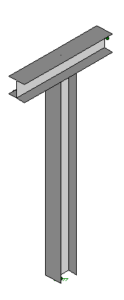# Steel Tee Support Design Mar 2019

Consider one of the most used pipe support structures — the tee support

Let’s just start with a basic design:

the tee is 8′ tall

bottom of base plate to top of steel beam

the tee beam is 4′ wide

the steel column and beam are W8x31 (A992)This size tee is one of the most sturdy that we will design.

any shorter and we would have used a W6x15 column and beam

any taller or wider and the deflections under load would be larger

Now let’s document the unit load deflections and the resulting k-factors

Axes and Boundary Conditions

Y is vertical
The tee is in the YZ plane
X is out of plane and
X is the longitudinal axis of the supported pipe
Column base is fixed

One kip load in each axis direction

Load point is at end point of the tee beam (2′ out from CL column)

X axis deflection = 2.39″; k-factor = 0.42 kips per inch (mostly beam rotation)
Y axis deflection = 0.03″; k-factor = 33.3 kips per inch
Z axis deflection = 0.12″; k-factor = 8.33 kips per inch

Notice the very low stiffness in response to lateral load Fx
This is because the wide flange column has relatively small torsional stiffness

One kip load in each axis direction

Load point is at intersection of tee column and beam

X axis deflection = 0.34″; k-factor = 2.94 kips per inch
Y axis deflection = 0.00″; k-factor = say ‘rigid’
Z axis deflection = 0.12″; k-factor = 8.33 kips per inch

Minimum loading for the tee is as follows:

tributary length: use at least 20′ (even if the spacing is less)

tributary width: use at least the beam width (consider future)

use a 40 psf gravity load (DL + FL) over the tributary area

use a friction coefficient, mu, of at least 40%

few lines on a tee; therefore, take no benefit for opposing friction loadings

use joint load Fx (LL) at end of beam of at least 0.5 kip

use joint load Fz (LL) at CL of beam of at least 0.5 kip

wind load basis: Fz (WL) at CL of beam

wind load basis: use at least largest diameter pipe + 10% of rack width

wind load basis: consider pipe insulation

wind load basis: use Cf of at least 0.70